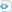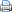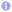# FizzBuzz or the beauty of compile-time calculations in C++17

FizzBuzz is a popular test that is commonly used in job interviews for software developers. In this test one is asked to write a function that prints the numbers from 1 to 100. But for multiples of three “Fizz” has to be printed instead of the number and for the multiples of five the word “Buzz” shall be printed. For numbers which are multiples of both three and five the word “FizzBuzz” results. This popular exercise has been solved in many different programming languages.

This is not really a challenging task. It is designed to divide candidates with virtually no programming expertise from those with some programming skills. In fact, the test requires very little programming skills and can be solved manually because it has no variable input parameters. An admissible solution would be to print just a fixed string:

 A straight-forward solution to FizzBuzz.#include <cstdlib>
#include <iostream>

int main() {
std::cout << "1\n2\nFizz\n4\nBuzz\nFizz\n7\n8\nFizz\nBuzz\n"
"11\nFizz\n13\n14\nFizzBuzz\n16\n17\nFizz\n19\nBuzz\n"
"Fizz\n22\n23\nFizz\nBuzz\n26\nFizz\n28\n29\nFizzBuzz\n"
"31\n32\nFizz\n34\nBuzz\nFizz\n37\n38\nFizz\nBuzz\n"
"41\nFizz\n43\n44\nFizzBuzz\n46\n47\nFizz\n49\nBuzz\n"
"Fizz\n52\n53\nFizz\nBuzz\n56\nFizz\n58\n59\nFizzBuzz\n"
"61\n62\nFizz\n64\nBuzz\nFizz\n67\n68\nFizz\nBuzz\n"
"71\nFizz\n73\n74\nFizzBuzz\n76\n77\nFizz\n79\nBuzz\n"
"Fizz\n82\n83\nFizz\nBuzz\n86\nFizz\n88\n89\nFizzBuzz\n"
"91\n92\nFizz\n94\nBuzz\nFizz\n97\n98\nFizz\nBuzz\n";
return EXIT_SUCCESS;
}

This solution is easy to program.  It has the nice feature, that actually nothing is computed at run-time. The whole string is known at compile-time. Coming up with such a solution is, however, tedious. What if we increase the upper limit from 100 to, let’s say, 1000?

Luckily, the compiler can do this job for us, at least in C++, and employing C++17 this becomes particularly easy. In the following program fizzbuzz_helper template classes are defined which carry a string array which contains either the string representation of a number or “Fizz” or “Buzz” or “FizzBuzz”, depending on the template parameter. This is pretty standard template programing stuff with C++11 or later. Beauty comes in in the concatenate const-expression function, which concatenates two arrays at compile-time. Due to some limitations of the array template class this is not possible in C++14 or earlier. At least not in such a clear and straight-forward way. The main routine, finally, just prints out the large string, which has been built by the compiler. You may check that the executable contains just the string, which is printed on the screen, via the strings utility on unix-like systems. Note that all class variables are declared as static constexpr to enforce compile-time calculation of the string value for initialization.

 Compile-time solution of FizzBuzz.#include <cstdlib>
#include <iostream>
#include <array>

// concatenate two arrays at compile-time
template<typename T, std::size_t N1, std::size_t N2>
constexpr std::array<T, N1+N2> concatenate(const std::array<T, N1> &lhs,
const std::array<T, N2> &rhs) {
std::array<T, N1+N2> result{};
std::size_t index{0};
for (std::size_t i{0}; i<lhs.size(); ++i, ++index)
result[index]=lhs[i];
for (std::size_t i{0}; i<rhs.size(); ++i, ++index)
result[index]=rhs[i];
return result;
}

// convert a 4-digit integer to a character string/array
template<std::size_t I>
struct int_to_str {
static_assert(I<=9999);
static constexpr char digits[]{"0123456789"};
static constexpr std::array<char, 5> str{ I>=1000 ? digits[(I/1000)%10] : ' ',
I>=100 ? digits[(I/100)%10] : ' ',
I>=10 ? digits[(I/10)%10] : ' ',
digits[(I/1)%10], '\n' };
};

// convert integer parameter I to string unless I can be devided by 3 or 5
// special cases where I can be divided 3 or 5 are handled via partial
// template specialization below
template<int I, bool div3=I%3==0, bool div5=I%5==0>
struct fizzbuzz_helper : int_to_str<I> {
};

template<int I>
struct fizzbuzz_helper<I, true, false> {
static constexpr std::array<char, 5> str{ 'F', 'i', 'z', 'z', '\n' };
};

template<int I>
struct fizzbuzz_helper<I, false, true> {
static constexpr std::array<char, 5> str{ 'B', 'u', 'z', 'z', '\n' };
};

template<int I>
struct fizzbuzz_helper<I, true, true> {
static constexpr std::array<char, 9> str{ 'F', 'i', 'z', 'z', 'B', 'u', 'z', 'z', '\n' };
};

// all Fizz-Buzz-integer strings are concatenated for all integers
// running from 1 to I via a recursive template definition
template<int I>
struct fizzbuzz {
static constexpr auto str{concatenate(fizzbuzz<I-1>::str, fizzbuzz_helper<I>::str)};
};

// stop the recursion via template specialization for I=1
template<>
struct fizzbuzz<1> {
static constexpr auto str{fizzbuzz_helper<1>::str};
};

int main() {
// print the compile-time concatenated Fizz-Buzz-integer string
//    1
//    2
// Fizz
//    4
// Buzz
// Fizz
//    7
//    8
// Fizz
// Buzz
//   11
// Fizz
//   13
//   14
// FizzBuzz
//   16
// ...
for (auto x: fizzbuzz<100>::str)
std::cout << x;
return EXIT_SUCCESS;
}